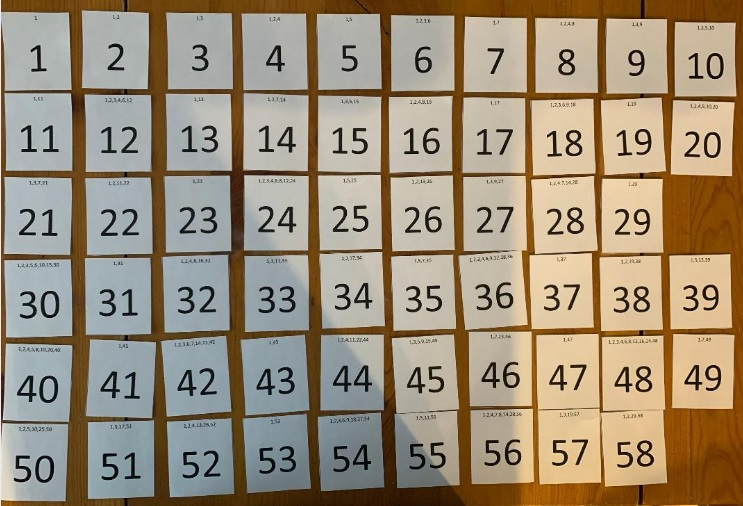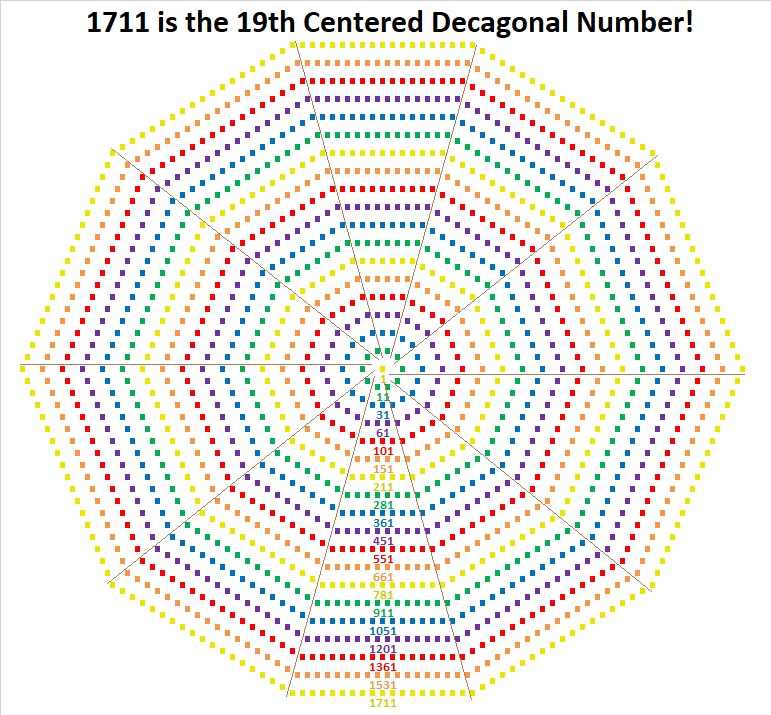1711 is a Triangular Number So The Taxman Wants His Share

Today’s Puzzle:

Imagine this puzzle is made up of 58 envelopes, each containing the amount of money printed in bold type on its front. You can’t take any of the envelopes without the Taxman also taking a share. The Taxman will take EVERY available envelope that has a factor of the number you take on it. When you have taken all the cash you can, the Taxman gets ALL the leftover cash, and the game is over. You want the Taxman to get as little money as possible.

How much money is at stake? (58 × 59)/2 = 1711. That means 1711 is a triangular number, the sum of all the numbers from 1 to 58. Thus, the total amount of money you will be splitting with the Taxman is 1711.

To play this game, you can print the cards from this excel file: Taxman & 1537-1544. The factors of a number is printed in small type at the top of its card.Here below I show the order I selected the cards when I played. For example, I took 53, and all the Taxman got was 1. I took 49, and the only card left for the Taxman to take was 7, and so forth. The last card I could take was 42, and the Taxman got 21, but since I couldn’t take anymore cards, the Taxman also got 31, 34, 37, 41, 43, and 47.To win the game, you must get over half of the 1711 cash, but of course, you will want the Taxman to get much less than nearly half the money.

I didn’t want to make a long addition problem to find out how much I kept, so I came up with an easier strategy: I pushed the Taxman’s share to the side, and looked for ways to make 100 from my cards. (It is almost the 100th day of school, after all.) I found 9 ways to make 100, so I clearly kept more than half of the 1711.I added that final 53 + 50 + 39 + 36 in my head by thinking that if I took 3 away from 53 and gave it to the 36, it would be the same as 50 + 50 + 39 + 39, a fairly easy sum.

Factors of 1711:

• 1711 is a composite number.
• Prime factorization: 1711 = 29 × 59.
• 1711 has no exponents greater than 1 in its prime factorization, so √1711 cannot be simplified.
• The exponents in the prime factorization are 1 and 1. Adding one to each exponent and multiplying we get (1 + 1)(1 + 1) = 2 × 2 = 4. Therefore 1711 has exactly 4 factors.
• The factors of 1711 are outlined with their factor pair partners in the graphic below.

1711 is a Shape-Shifting Number:

• 1711 is the 58th Triangular Number because (58·59)/2 = 1711.
• It is the 20th Centered Nonagonal Number because it is one more than nine times the 19th triangular number: 9(19·20)/2 + 1 =1711. AND
• It is the 19th Centered Decagonal Number because it is one more than 10 times the 18th triangular number: 10(18·19)/2 +1 = 1711.

That last figure I’ve illustrated below with ten triangles circled around the center dot:Now get this: Not only is 1711 the 19th Centered Decagonal Number, but similar-looking 17111 is the 59th Centered Decagonal Number! (A mere coincidence, but the 59th is even cooler because 59·29 = 1711.)